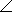# Electrical Engineering - RL Circuits - Discussion

### Discussion :: RL Circuits - General Questions (Q.No.14)

14.

A 47resistor is in series with an inductive reactance of 120across an ac source. The impedance, expressed in polar form, is

 [A]. 4768.6°[B]. 12068.6°[C]. 12931.4°[D]. 12968.6°Explanation:

No answer description available for this question.

 Arjun said: (Jan 4, 2012) Magnitude=( r^2+ x^2)^12 tan(phase angle) = 120/47 So ans is magnitude*phase angle = Ans D.

 Priya said: (Mar 11, 2015) Z = R+jXL. = 47+j120. In polar form: = 128.87 angle 68.61.

 D Gomathi said: (Dec 24, 2015) I don't understand please explain.

 Gnani said: (Feb 5, 2016) R = 47; XL = 120 then R+jXL = R^2+X^2. = 47^2 + 120^2. = 128.87. tan^-1 (XL/R) = tan^-1 (120/47) = 68.8.

 B Sharath Kumar said: (Jan 31, 2017) Please, anyone explain how to calculate tan inverse of 120/40 without calculator?

 Buddhadeb Singha said: (Dec 29, 2017) How to calculate? 47^2 + 120^2= 128.87.

 Raj said: (Jan 30, 2018) Z= R+jXL. = (47^2+120^2)1^1/2. = 128.87. = tan^-1(XL/R)=68.8.